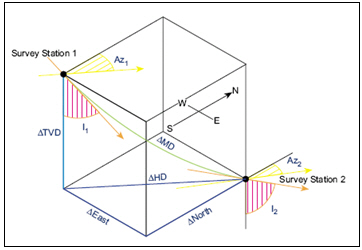# Dogleg Severity Calculation based on Radius of Curvature Method

Dogleg severity (DLS) is a normalized estimation, normally described in degrees per 100 feet or degree per 30 meters, of the overall well bore curvature between two consecutive directional surveys. Regarding a planned well path, dogleg severity may be synonymous about build and/or turn. The following formula provides dogleg severity in degrees/100 ft  based on the Radius of Curvature Method.Dogleg severity (DLS) = {cos-1 [(cos I1 x cos I2) + (sin I1 x sin I2) x cos (Az2 – Az1)]} x (100 ÷ MD)

Where;

DLS = dogleg severity in degrees/l00 ft

MD = Measured Depth between survey points in ft

I1 = Inclination (angle) at upper survey in degrees

I2 = Inclination (angle) at lower in degrees

Az1= Azimuth direction at upper survey

Az2 = Azimuth direction at lower survey

Example for dogleg severity based on Radius of Curvature Method

Survey 1

Depth = 7500 ft

Inclination = 45 degree (I1)

Azimuth = 130degree (Az1)

Survey 2

Depth = 7595 ft

Inclination = 52 degree (I2)

Azimuth = 139 degree (Az2)

Dogleg severity (DLS) = {cos-1 [(cos 45 x cos 52) + (sin 45 x sin 52) x cos (139 – 130)]} x (100 ÷ 95)

Dogleg severity (DLS) =  10.22 degree/100 ft

Please find the Excel sheet used to calculate dogleg severity based on Radius of Curvature Method.

Share the joyWorking in the oil field and loving to share knowledge.

### 3 Responses to Dogleg Severity Calculation based on Radius of Curvature Method

1.cna training says:

found your site on del.icio.us today and really liked it.. i bookmarked it and will be back to check it out some more later

2.Slickliner says:

and this is the same tangential method. Well this is the correct Tangential Method. It just a matter of simplified Trigonometric Law. cos (B-A) = sinA*sinB + cosA*cosB.
Radius Curvature Method is a way complicated than showed above.

This site uses Akismet to reduce spam. Learn how your comment data is processed.